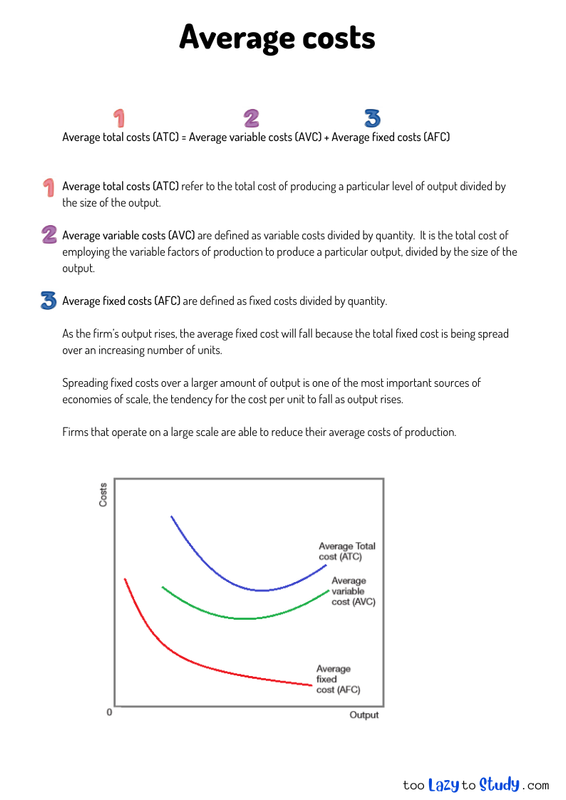top of page

# Economics explained

Category:

Firm's cost structure# 👑 Economics Revision Notes

The secret to scoring awesome grades in economics is to have corresponding awesome notes.

A common pitfall for students is to lose themselves in a sea of notes: personal notes, teacher notes, online notes textbooks, etc... This happens when one has too many sources to revise from! Why not solve this problem by having one reliable source of notes? This is where we can help.

What makes TooLazyToStudy notes different?

Our notes:
• are clear and concise and relevant
• is set in an engaging template to facilitate memorisation
• cover all the important topics in the O level, AS level and A level syllabus
• are editable, feel free to make additions or to rephrase sentences in your own words!

Looking for live explanations of these notes? Enrol now for FREE tuition!

Average total costs (ATC) = Average variable costs (AVC) + Average fixed costs (AFC)

Average total costs (ATC) refer to the total cost of producing a particular level of output divided by the size of the output.

Average variable costs (AVC) are defined as variable costs divided by quantity. It is the total cost of employing the variable factors of production to produce a particular output, divided by the size of the output.

Average fixed costs (AFC) are defined as fixed costs divided by quantity.

As the firm’s output rises, the average fixed cost will fall because the total fixed cost is being spread over an increasing number of units.

Spreading fixed costs over a larger amount of output is one of the most important sources of economies of scale, the tendency for the cost per unit to fall as output rises.

Firms that operate on a large scale are able to reduce their average costs of production.

bottom of page